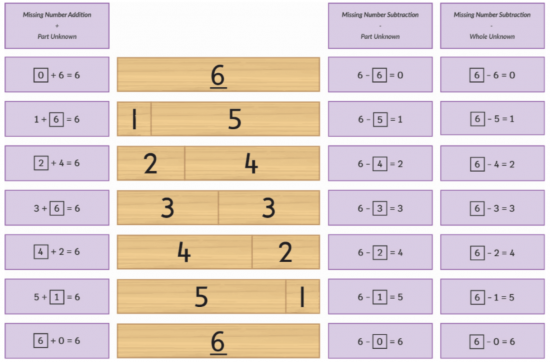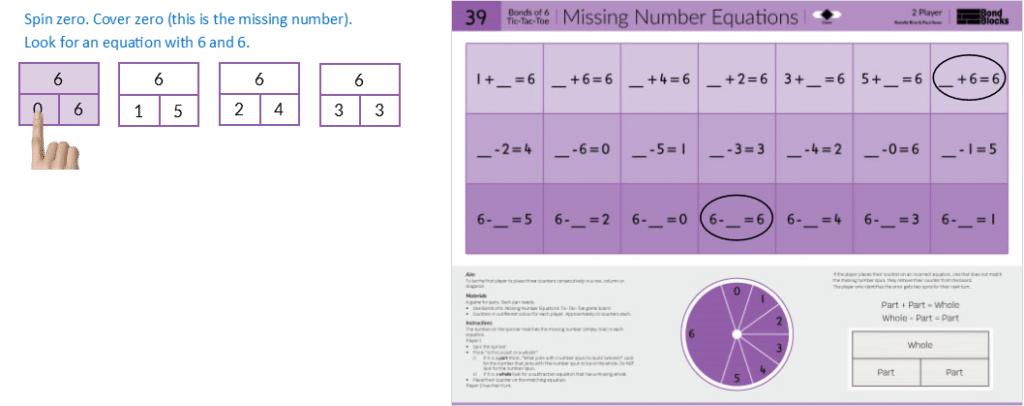## 39) Missing Number Equations

Bonds of 6, 7, 8, 9: Tic-Tac-Toe### Mathematics

Develop the concept of using part-part-whole, to calculate an unknown part or whole, in addition and subtraction equations, for a specified whole (6, 7,8 or 9).

This is a complex concept that has been highly scaffolded in previous Missing Number Activities.

• First, students need to identify if the unknown number could be in the position of a part or a whole.
• Unknown parts can be calculated by either adding on or subtracting. For example, 6-?=2 can be solved by
(i) adding on to the known part to reach the whole, 2+?=6 or
(ii) making a subtraction equation where the unknown part is in the answer position, 6-2=?
• Unknown wholes are calculated by adding the known parts.

The unknown numbers in Activity 39 are placed in random order, within each row. In previous Missing Number Core Activities, related bonds were grouped together in rows and the unknown number was always a part. Activity 39 is more difficult because (i) it introduces concept of an unknown whole in a subtraction equation and, (ii) within each row the unknown numbers are in random order.

• Row One contains addition equations with an unknown part.
• Row Two contains subtraction equations with an unknown whole.
• Row Three contains subtraction equations with an unknown part (that is not in the answer position).

### Language

• subtraction as ‘subtract’. Read aloud as “(Whole) subtract (part) equals (part)”.
• equals
• part-part-whole
• missing part solved by thinking, “What joins with (number spun) to build 20?”
• missing whole solved by thinking, “Look for a subtraction equation what has a missing whole.”
• The activity board uses the word “missing”. The word “unknown” is introduced when the Missing Number Sorting Cards are used.## Differentiation

### A little easier

##### Card sort

Click this link to download the Missing Number Equations Card Sort for the relevant whole (6, 7, 8 or 9) from the top of this page. Each card is one of the equations from the Missing Number Equations game board. Instruct students to:

• Cut out each card.
• Mix the cards.
• Sort the cards into three groups using the diagram heading cards:
• subtraction: one part unknown
• subtraction: whole unknown

After sorting the cards. Instruct students to:

• Build a two-part bond wall for the relevant whole. Use two of each block from 1, to the whole.
• Start with the addition cards. Select one row from the bond wall. Search the addition cards for related equations. Fill in the unknown part and place these cards next to the related Bond Blocks. Repeat for each row until all the missing number addition cards have been filled in and placed next to the related row of Bond Blocks.
• Repeat for the subtraction cards with an unknown part.
• Repeat for the subtraction cards with an unknown whole.
• Discuss strategies for calculating the unknown, including the importance of first identifying if it is a missing part or whole.##### Build a wall
• Build a two-part bond wall for the relevant whole. Use one of each block from 1 to the whole. For the whole of 6 use one additional 3 block to build double 3. For the whole of 8 use one additional 4 block for double 4. Place this next to the activity board.
• Place the activity board in a write and wipe sleeve. Circle all the subtraction equations where the missing number is in the whole position.
• Use both of these scaffolds to either play or solve each missing number equation. Record this missing number on the write and wipe sleeve using a dry erase marker.##### Part-part-whole desk visual
• Click to download the Part-Part-Whole Desk Visual for the relevant whole.
• Place this next to the activity board.
• Flick the spinner then use the part-part-whole diagram to identify what to look for on the activity board.

For example, spinning 4For example, spinning zeroFor example, spinning 6### Progression

In the next activity students solve word problems, across of range of different types, for the wholes of 7, 8, 9 and 10.The unknowns have been placed in a variety of of positions. Go to

##### Activity 40

Bonds of 6, 7, 8, 9: Word Problems, Wholes to 10